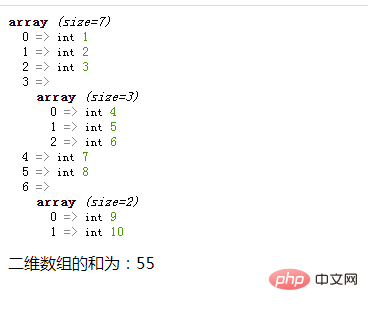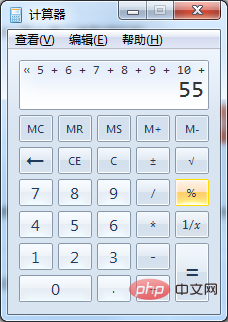# php怎么求二维数组的和php求二维数组和的方法

1、使用foreach语句循环遍历二维数组的外层数组元素

```foreach(\$arr as \$v){
//循环体代码
}```

2、在循环体，使用is_array()函数判断外层元素是否为数组类型，并利用“+=”运算符求和

• 如果不是数组类型，则将不是的外层元素相加求和

• 如果是数组类型，则使用array_sum()函数计算该内层数组中所有元素的和，并与外层元素和相加在一起即可。

```if(is_array(\$v)){
\$sum+=array_sum(\$v);
}else{
\$sum+=\$v;
}```

```<?php
\$arr = array(1,2,3,array(4,5,6),7,8,array(9,10));
var_dump(\$arr);
\$sum=0;
foreach(\$arr as \$v){
if(is_array(\$v)){
\$sum+=array_sum(\$v);
}else{
\$sum+=\$v;
}
}
echo "二维数组的和为：".\$sum;
?>```1/1